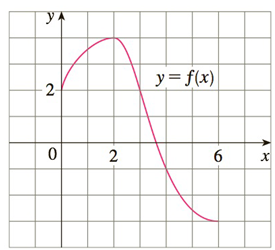Chapter 4.R, Problem 1E

Chapter
Section
Textbook Problem

# Use the given graph of f to find the Riemann sum with six subintervals. Take the sample points to be (a) left endpoints and (b) mid points. In each case draw a diagram and explain what the Riemann sum represents.To determine

a)

To find:

The Riemann sum for the left endpoints with six subintervals

Explanation

1) Concept:

Definition of the Riemann sum:

i=1nfxi* x

is called as the Riemann sum

Where,

x= b-an,    xi=a+i x,    & xi*xi-1, xi

Riemann sum is the sum, of the areas of the rectangles that lie above the x-axis and the negative of the areas of the rectangles that lie below the x-axis

2) Calculation:

First, write the expression of the Riemann sum for left endpoints

Six subintervals are given, that means, n=6

By definition of Riemann sum,

i=1nfxi x=i=16fxi x

So now calculate

To determine

b)

To find:

The Riemann sum for midpoints with six subintervals

### Still sussing out bartleby?

Check out a sample textbook solution.

See a sample solution

#### The Solution to Your Study Problems

Bartleby provides explanations to thousands of textbook problems written by our experts, many with advanced degrees!

Get Started

#### Evaluate the integral. 1/21/241x2dx

Single Variable Calculus: Early Transcendentals, Volume I

#### Expand each expression in Exercises 122. (x22x+1)2

Finite Mathematics and Applied Calculus (MindTap Course List)

#### In Exercises 73-80, find the indicated limits, if they exist. 76. limx2x2+3x+1x4x2

Applied Calculus for the Managerial, Life, and Social Sciences: A Brief Approach

#### Which is the largest? a) f(a) b) f(b) c) f(c) d) cannot tell from information given

Study Guide for Stewart's Single Variable Calculus: Early Transcendentals, 8th

#### The graph of x = cos t, y = sin2 t is:

Study Guide for Stewart's Multivariable Calculus, 8th